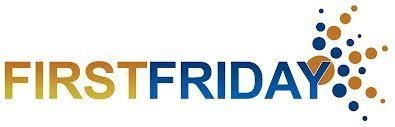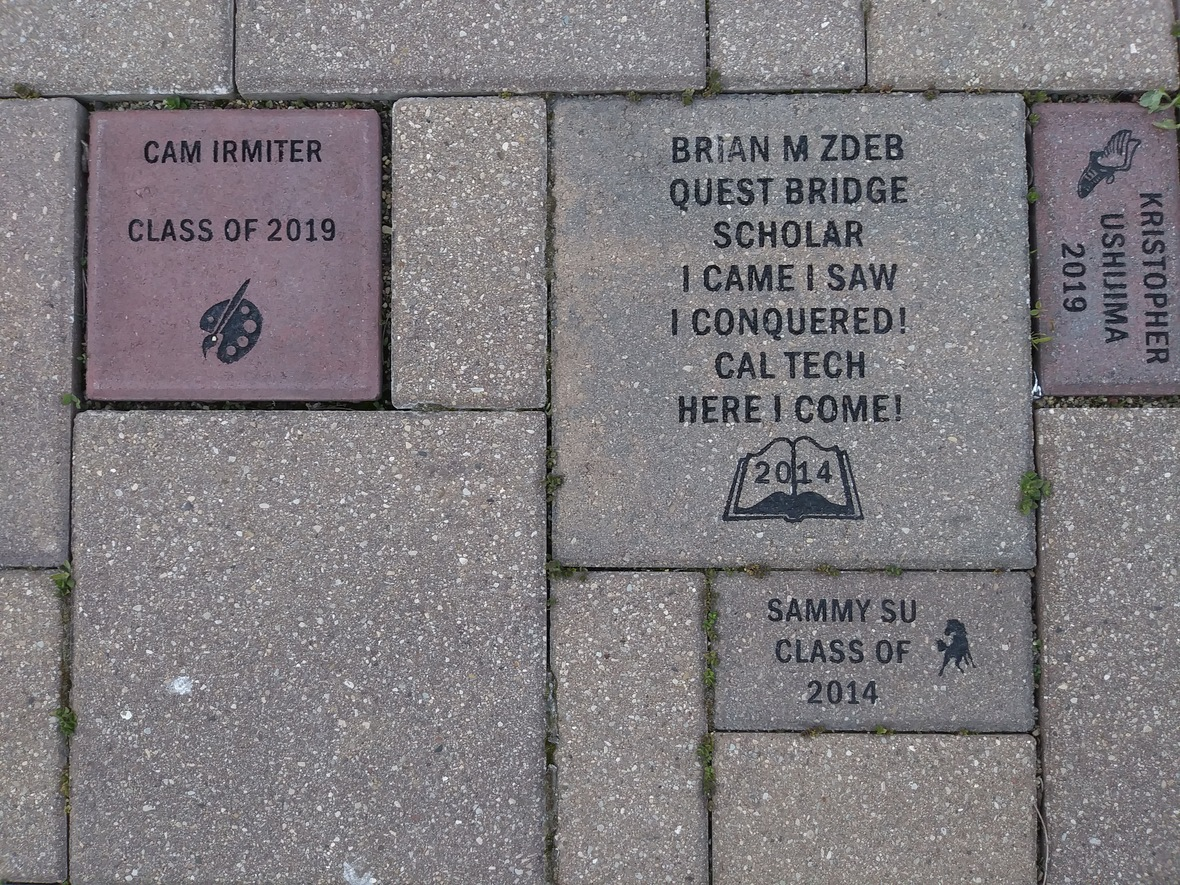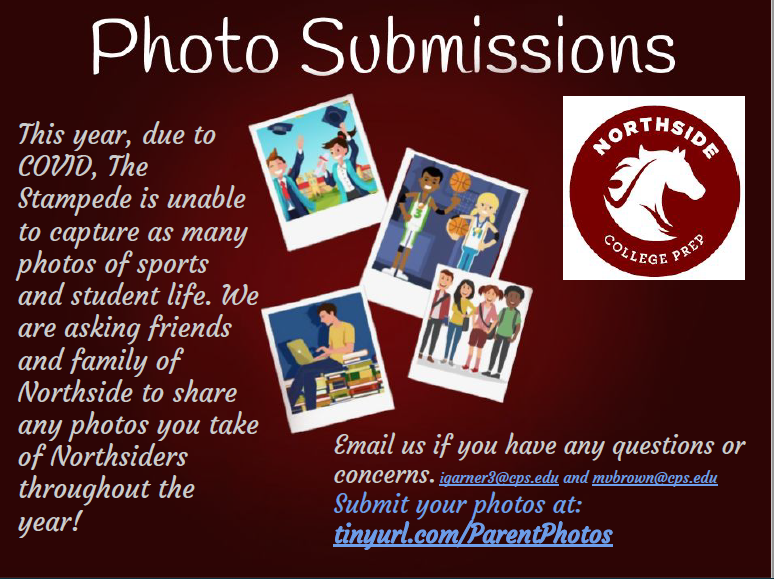## Sunday, January 31, 2021# Upcoming Important Dates

Monday, February 1 - AP Payment Deadline
Friday, February 5 - Virtual First Friday
Monday, February 8 - NCP College Night (Rescheduled)

 table div table+table+table+table+table div table{width:100%;padding:0}table div table+table+table+table+table div table img{width:96.23%;padding:0;float:none}table div table+table+table+table+table div table td{width:100%;padding:0 1.88% 18px}/* styles */▪ Annual Appeal Update
▪ AP Exam Payment Info: Deadline to Pay is TOMORROW, February 1, 2021
▪ Virtual College Planning Night Rescheduled: Monday, February 8 at 6:00 pm
▪ Summer Pre-College Program Fair
▪ Virtual Fair Dates
▪ College Representative Visits
▪ Verto Education Virtual College Fair
▪ Available Now: Custom NCP Brick With Your Personalized Message for Your Student
▪ SAT Updates from College Board
▪ Counseling Department: ILP Naviance Curriculum
▪ Photo Submissions Requested for NCP Stampede!
▪ Yearbook Ads for Seniors Available
▪ Order Your 2020-2021 NCP Yearbook
▪ Ways for Parents to Stay in Touch
 ▪ Annual Appeal Update
 ▪ AP Exam Payment Info: Deadline to Pay is TOMORROW, February 1, 2021
 ▪ Virtual College Planning Night Rescheduled: Monday, February 8 at 6:00 pm
 ▪ Summer Pre-College Program Fair
 ▪ Virtual Fair Dates
 ▪ College Representative Visits
 ▪ Verto Education Virtual College Fair
 ▪ Available Now: Custom NCP Brick With Your Personalized Message for Your Student
 ▪ SAT Updates from College Board
 ▪ Counseling Department: ILP Naviance Curriculum
 ▪ Photo Submissions Requested for NCP Stampede!
 ▪ Yearbook Ads for Seniors Available
 ▪ Order Your 2020-2021 NCP Yearbook
 ▪ Ways for Parents to Stay in Touch
 table div table+table+table+table+table+table+table div table{width:100%;padding:0}table div table+table+table+table+table+table+table div table img{width:96.23%;padding:0;float:none}table div table+table+table+table+table+table+table div table td{width:100%;padding:0 1.88% 18px}/* styles */# THANK YOU to the approximately 190 families that donated a combined total of \$113,000 to our Annual Appeal

Your donations will make a difference as we support our Northside school community, most importantly our students, through clubs, athletics, counseling, security, and more. It takes everyone pitching in to make it all work and we are grateful for your investment through Friends of Northside (FON).

If you didn't get a chance to get your gift in for the appeal, you can still donate below.

Contact Friends of Northside at info@friendsofnorthside.org with any questions.

 table div table+table+table+table+table+table+table+table+table+table+table div table{width:100%;padding:0}table div table+table+table+table+table+table+table+table+table+table+table div table img{width:96.23%;padding:0;float:none}table div table+table+table+table+table+table+table+table+table+table+table div table td{width:100%;padding:0 1.88% 18px}/* styles */# AP Exam Payment Info: Deadline to Pay is TOMORROW, February 1, 2021

Students currently enrolled in AP courses may now pay for their exams. AP exam fees have been added to student accounts in ASPEN. The amount shown is the exact amount owed for those exams specifically. Please follow the instructions below to make payments by Monday, February 1, 2021.

Students currently enrolled in AP courses may now pay for their exams. AP exam fees have been added to student accounts in ASPEN. The amount shown is the exact amount/total owed for those exams specifically.

## To Pay:

1. Click on Family.
2. Click on Transactions.
3. Click on Fees. There you should see the AP Exam(s) for your student.
4. Click on Pay Online. This will take you to the e-Pay website.
5. Select AP Classes/exam and the correct cost based on the total amount owed for AP exams in your ASPEN account.

At this time, we are not able to take cash or physical check payments. This is a CPS policy for this year.

The NEW AP PAYMENT deadline is Monday, February 1.
*Fees have been waived for students who have an approved fee waiver for the 2020-21 school year.

 table div table+table+table+table+table+table+table+table+table+table+table+table+table+table div table{width:100%;padding:0}table div table+table+table+table+table+table+table+table+table+table+table+table+table+table div table img{width:96.23%;padding:0;float:none}table div table+table+table+table+table+table+table+table+table+table+table+table+table+table div table td{width:100%;padding:0 1.88% 18px}/* styles */# First Friday Virtual Gathering

Friday, February 5
9:00 am Zoom gathering

Each month, NCP parents and guardians are invited to get together for a monthly coffee gathering. This month, join fellow NCP parents online. Stay for as long as your schedule allows.

## Join Zoom meeting

▪ Meeting ID: 848 7451 7349
▪ Passcode: 336446
▪ One tap mobile +13126266799,,84874517349#,,,,,,0#,,336446# US (Chicago)
 ▪ Meeting ID: 848 7451 7349
 ▪ Passcode: 336446
 ▪ One tap mobile +13126266799,,84874517349#,,,,,,0#,,336446# US (Chicago)
 table div table+table+table+table+table+table+table+table+table+table+table+table+table+table+table+table div table{width:100%;padding:0}table div table+table+table+table+table+table+table+table+table+table+table+table+table+table+table+table div table img{width:96.23%;padding:0;float:none}table div table+table+table+table+table+table+table+table+table+table+table+table+table+table+table+table div table td{width:100%;padding:0 1.88% 18px}/* styles */# Virtual College Planning Night Rescheduled: Monday, February 8 at 6:00 pm

Junior Students and Parents,

The counseling department has decided to reschedule the College Planning Night originally scheduled for Tuesday, February 2 to Monday, February 8.

The original date conflicts with the final exam week. If you already registered for the previous date, we received your registration and you do not need to complete the form again.

This year, we are offering the virtual College Planning presentation in English and Spanish to meet the needs of our families. Also, each counselor will facilitate the virtual presentation for their respective advisories. To identify your advisory or school counselor, refer to the list below:

▪ 200 - Mourtokokis
▪ 201 - Mourtokokis
▪ 202 - Mathews
▪ 203 - Mathews
▪ 204 - Mathews
▪ 205 - Hughes
▪ 206 - Hughes
▪ 207 - Hughes
▪ 208 - Medina/Moore
▪ 209 - Medina/Moore *Spanish Speaking Families - Medina
 ▪ 200 - Mourtokokis
 ▪ 201 - Mourtokokis
 ▪ 202 - Mathews
 ▪ 203 - Mathews
 ▪ 204 - Mathews
 ▪ 205 - Hughes
 ▪ 206 - Hughes
 ▪ 207 - Hughes
 ▪ 208 - Medina/Moore
 ▪ 209 - Medina/Moore *Spanish Speaking Families - Medina

During the virtual presentation, the counseling team will share an overview of the college admission process, application timeline, post-secondary resources, and information to assist your family with scheduling a college conference. Also, each family will receive access to the electronic College Planning Handbook, and parents will receive their registration code for Naviance, our college application management program.

Our goal is 100% attendance for juniors and their parents or guardians. College Planning Night will assist your family in successfully navigating the postsecondary process! This is an exciting time in your life and the NCP counseling team is prepared to assist you with your postsecondary journey.
If you have any questions or concerns, contact Ms. Moore at mcmoore6@cps.edu.

Sincerely,

The Counseling Department

# Summer Pre-College Program Fair

The Association for Pre-College Program Directors is hosting fairs featuring summer programs at leading colleges and universities. Current high school students, parents, and guardians are invited to register for the 2021 Summer Pre-College Virtual Program Fairs, beginning this month. Attendees will have the opportunity to connect with representatives from leading US colleges and universities to learn more about Summer 2021 academic programs for high school students.

Visit virtual booths, where you can ask questions via video or text chat with summer program representatives. View helpful webinars on how to choose a summer program, how to strengthen your application, how to make the most out of your summer program experience. Registration is FREE to all attendees. Limit one event registration per household.

## Virtual Fair Dates

Wednesday, February 3
Saturday, February 20

Click the following link https://www.summerstudyintheusa.org/virtualstudentfairs// to register.

# Verto Education Virtual College Fair

Wednesday, February 10
3:00 pm - 5:00 pm PT/ 6:00 pm - 8:00 pm ET

Register HERE!

Join Verto Education for our first national college fair of 2021, with 50+ Colleges and Universities, and national education experts! We are limiting attendance for each fair to 5,000 students, parents, and counselors. Come meet with college admissions counselors from top colleges and universities, hear a Keynote and Q&A from national experts, hear from current college students about their experiences, and attend college readiness workshops!

Come ready with questions for the admissions representatives at our wonderful participating schools!

List of Participating Schools:

▪ Arizona State University
▪ Belhaven University
▪ Berry College
▪ Binghamton University
▪ Bucknell University
▪ Butler University
▪ Case Western Reserve University
▪ Catholic University
▪ Chatham University
▪ Clark University
▪ College of Wooster
▪ Cornell College
▪ Denison University
▪ DePauw University
▪ Goucher College
▪ Hanover College
▪ High Point University
▪ Illinois Institute of Technology
▪ Loyola University Chicago
▪ Loyola University of Maryland
▪ Lynn University
▪ Merrimack College
▪ Mills College
▪ Moravian College
▪ Muhlenberg College
▪ Northern Michigan University
▪ Ohio University
▪ Ohio Wesleyan University
▪ Pace University
▪ Portland State University
▪ Prescott College
▪ Regis University
▪ Richard Bland College
▪ Saint Louis University
▪ Saint Michael's College
▪ St. Mary’s College of Maryland
▪ Temple University
▪ University of Dayton
▪ University of Denver
▪ University of Oregon
▪ University of South Florida
▪ University of Southern Maine
▪ University of Tennessee
▪ University of Vermont
▪ Valparaiso University
▪ Verto Education
▪ Virginia Polytechnic Institute and State University
▪ Washington & Jefferson College
 ▪ Arizona State University
 ▪ Belhaven University
 ▪ Berry College
 ▪ Binghamton University
 ▪ Bucknell University
 ▪ Butler University
 ▪ Case Western Reserve University
 ▪ Catholic University
 ▪ Chatham University
 ▪ Clark University
 ▪ College of Wooster
 ▪ Cornell College
 ▪ Denison University
 ▪ DePauw University
 ▪ Goucher College
 ▪ Hanover College
 ▪ High Point University
 ▪ Illinois Institute of Technology
 ▪ Loyola University Chicago
 ▪ Loyola University of Maryland
 ▪ Lynn University
 ▪ Merrimack College
 ▪ Mills College
 ▪ Moravian College
 ▪ Muhlenberg College
 ▪ Northern Michigan University
 ▪ Ohio University
 ▪ Ohio Wesleyan University
 ▪ Pace University
 ▪ Portland State University
 ▪ Prescott College
 ▪ Regis University
 ▪ Richard Bland College
 ▪ Saint Louis University
 ▪ Saint Michael's College
 ▪ St. Mary’s College of Maryland
 ▪ Temple University
 ▪ University of Dayton
 ▪ University of Denver
 ▪ University of Oregon
 ▪ University of South Florida
 ▪ University of Southern Maine
 ▪ University of Tennessee
 ▪ University of Vermont
 ▪ Valparaiso University
 ▪ Verto Education
 ▪ Virginia Polytechnic Institute and State University
 ▪ Washington & Jefferson College
 table div table+table+table+table+table+table+table+table+table+table+table+table+table+table+table+table+table+table+table div table{width:100%;padding:0}table div table+table+table+table+table+table+table+table+table+table+table+table+table+table+table+table+table+table+table div table img{width:96.23%;padding:0;float:none}table div table+table+table+table+table+table+table+table+table+table+table+table+table+table+table+table+table+table+table div table td{width:100%;padding:0 1.88% 18px}/* styles */Struggling to find one last gift for your child this year? Consider buying a custom NCP Brick. Your brick, engraved with a personal message, will become a permanent part of the Northside College Prep Celestial Garden Walkway.

Your brick may be purchased in three sizes:

▪ 4" x 8" = \$50
▪ 8" x 8" = \$100
▪ 12" x 12" = \$150

Friends of Northside will provide a gift certificate acknowledging the purchase that can be given during the holiday season. Janet Jasmer will assist with the process and can answer any questions at jjasmer@atproperties.com.

 table div table+table+table+table+table+table+table+table+table+table+table+table+table+table+table+table+table+table+table+table+table+table div table{width:100%;padding:0}table div table+table+table+table+table+table+table+table+table+table+table+table+table+table+table+table+table+table+table+table+table+table div table img{width:96.23%;padding:0;float:none}table div table+table+table+table+table+table+table+table+table+table+table+table+table+table+table+table+table+table+table+table+table+table div table td{width:100%;padding:0 1.88% 18px}/* styles */# Any Questions About NCP Communications Sent Via CNXT?

If you are having problems with SchoolCNXT, please contact the main office from 7:30 am - 3:15 pm at 773-534-3954, or you can email the NCP school clerk, Mary Grasz, at mtgrasz@cpu.edu.

NCP uses SchoolCNXT to communicate important information to parents and the school community. All families should have received a text message and/or email communication about signing up. If you did not receive that communication or need access to your student's account, you can gain access by using this tool on Northside's website.

 table div table+table+table+table+table+table+table+table+table+table+table+table+table+table+table+table+table+table+table+table+table+table+table+table+table div table{width:100%;padding:0}table div table+table+table+table+table+table+table+table+table+table+table+table+table+table+table+table+table+table+table+table+table+table+table+table+table div table img{width:96.23%;padding:0;float:none}table div table+table+table+table+table+table+table+table+table+table+table+table+table+table+table+table+table+table+table+table+table+table+table+table+table div table td{width:100%;padding:0 1.88% 18px}/* styles */# College Representative Visits

The NCP Counseling Department is offering virtual postsecondary visits for Northside Prep students and parents. We are offering virtual postsecondary visits to ensure all families have the opportunity to explore various postsecondary pathways, engage with the postsecondary representatives, and obtain answers to pertinent questions or concerns. Virtual postsecondary visits are offered after school from 3:15 pm - 4:00 pm and during Flex Blocks 1-4 on Wednesdays.

To date, college, military, and GAP Year representatives are scheduled to connect with NCP students virtually, and the list is growing every day. Click HERE to view the list of upcoming and past postsecondary visits. Also, check out the messages from the admissions representatives and the additional resources they shared during their visits.

*Please note, all virtual visits will be conducted via CPS Google Meets and no email addresses outside CPS will be permitted into the virtual postsecondary visits. To protect the privacy and safety of students, parents will only be permitted to access virtual visits if their respective student is present and the student will be required to log in via their CPS Gmail account. All postsecondary visits will be managed and monitored by an NCP staff member.

 table div table+table+table+table+table+table+table+table+table+table+table+table+table+table+table+table+table+table+table+table+table+table+table+table+table+table+table div table{width:100%;padding:0}table div table+table+table+table+table+table+table+table+table+table+table+table+table+table+table+table+table+table+table+table+table+table+table+table+table+table+table div table img{width:96.23%;padding:0;float:none}table div table+table+table+table+table+table+table+table+table+table+table+table+table+table+table+table+table+table+table+table+table+table+table+table+table+table+table div table td{width:100%;padding:0 1.88% 18px}/* styles */# CPS Scholarship Notifications and College Events Around the City

For more details about upcoming events and to access the most recent updates, click HERE to view the College Calendar on the Northside Prep Counseling website. Also, for additional scholarship opportunities, click HERE to visit the Northside College Prep Counseling page.

 table div table+table+table+table+table+table+table+table+table+table+table+table+table+table+table+table+table+table+table+table+table+table+table+table+table+table+table+table+table div table{width:100%;padding:0}table div table+table+table+table+table+table+table+table+table+table+table+table+table+table+table+table+table+table+table+table+table+table+table+table+table+table+table+table+table div table img{width:96.23%;padding:0;float:none}table div table+table+table+table+table+table+table+table+table+table+table+table+table+table+table+table+table+table+table+table+table+table+table+table+table+table+table+table+table div table td{width:100%;padding:0 1.88% 18px}/* styles */# SAT Updates from the College Board

As students and colleges adapt to new realities and changes in the college admissions process, the College Board is making sure our programs adapt with them.

The pandemic accelerated a process already underway at the College Board to simplify our work and reduce demands on students. As part of this process, we're making substantial investments in the SAT® Suite of Assessments and in tools to help colleges connect with students. We are providing three updates today and will continue to consult with our members on this ongoing work. Click here for more details about the updates.

 table div table+table+table+table+table+table+table+table+table+table+table+table+table+table+table+table+table+table+table+table+table+table+table+table+table+table+table+table+table+table+table div table{width:100%;padding:0}table div table+table+table+table+table+table+table+table+table+table+table+table+table+table+table+table+table+table+table+table+table+table+table+table+table+table+table+table+table+table+table div table img{width:96.23%;padding:0;float:none}table div table+table+table+table+table+table+table+table+table+table+table+table+table+table+table+table+table+table+table+table+table+table+table+table+table+table+table+table+table+table+table div table td{width:100%;padding:0 1.88% 18px}/* styles */# Counseling Department: ILP Naviance Curriculum

Northside Prep Families,

The CPS Office of School Counseling and Postsecondary Advising has created quarterly newsletters to continue to engage and support students and parents with their postsecondary planning, including: self-discovery, academic planning, and career and college exploration. To view the newsletters for each grade level click on the links below. To access the counseling department college planning curriculum, students and parents can log into their Naviance account here to review and complete each task.

 table div table+table+table+table+table+table+table+table+table+table+table+table+table+table+table+table+table+table+table+table+table+table+table+table+table+table+table+table+table+table+table+table+table div table{width:100%;padding:0}table div table+table+table+table+table+table+table+table+table+table+table+table+table+table+table+table+table+table+table+table+table+table+table+table+table+table+table+table+table+table+table+table+table div table img{width:96.23%;padding:0;float:none}table div table+table+table+table+table+table+table+table+table+table+table+table+table+table+table+table+table+table+table+table+table+table+table+table+table+table+table+table+table+table+table+table+table div table td{width:100%;padding:0 1.88% 18px}/* styles */# Photo Submissions Requested for NCP Stampede!# 2020-2021 Yearbook Ads for Seniors Now Available

Seniors now have the opportunity to place ads in the 2020-2021 yearbook by visiting the yearbook website. After clicking on "continue", click on "start your ad" to begin.

 table div table+table+table+table+table+table+table+table+table+table+table+table+table+table+table+table+table+table+table+table+table+table+table+table+table+table+table+table+table+table+table+table+table+table+table+table+table div table td,table.module-36{width:100%;padding:0}table div table+table+table+table+table+table+table+table+table+table+table+table+table+table+table+table+table+table+table+table+table+table+table+table+table+table+table+table+table+table+table+table+table+table+table+table+table div table{width:100%;float:none;margin-left:auto;margin-right:auto;padding:0}table div table+table+table+table+table+table+table+table+table+table+table+table+table+table+table+table+table+table+table+table+table+table+table+table+table+table+table+table+table+table+table+table+table+table+table+table+table div table a{border:0 none;text-decoration:none}table div table+table+table+table+table+table+table+table+table+table+table+table+table+table+table+table+table+table+table+table+table+table+table+table+table+table+table+table+table+table+table+table+table+table+table+table+table div table img{width:100%!important;border:0 none;text-decoration:none}/* styles */

# Order a Yearbook Today!

Interested in purchasing a copy of the 2020-2021 Stampede, NCP's yearbook? Click HERE to start your order! And if you didn't get a chance to purchase a copy of the 2019-2020 yearbook, you now have a change to buy one of those, too! Just email Ms. McCreedy at mkmccreedy@cps.edu after you've completed your 2020-2021 order.

Copies are \$45 until March 1 and \$55 afterwards.table div table+table+table+table+table+table+table+table+table+table+table+table+table+table+table+table+table+table+table+table+table+table+table+table+table+table+table+table+table+table+table+table+table+table+table+table+table+table+table+table div table{width:100%;padding:0}table div table+table+table+table+table+table+table+table+table+table+table+table+table+table+table+table+table+table+table+table+table+table+table+table+table+table+table+table+table+table+table+table+table+table+table+table+table+table+table+table div table img{width:96.23%;padding:0;float:none}table div table+table+table+table+table+table+table+table+table+table+table+table+table+table+table+table+table+table+table+table+table+table+table+table+table+table+table+table+table+table+table+table+table+table+table+table+table+table+table+table div table td{width:100%;padding:0 1.88% 18px}/* styles */# Do you have news or team results to share with the Northside parent community?

 table div table+table+table+table+table+table+table+table+table+table+table+table+table+table+table+table+table+table+table+table+table+table+table+table+table+table+table+table+table+table+table+table+table+table+table+table+table+table+table+table+table+table+table div table{width:100%;padding:0}table div table+table+table+table+table+table+table+table+table+table+table+table+table+table+table+table+table+table+table+table+table+table+table+table+table+table+table+table+table+table+table+table+table+table+table+table+table+table+table+table+table+table+table div table img{width:96.23%;padding:0;float:none}table div table+table+table+table+table+table+table+table+table+table+table+table+table+table+table+table+table+table+table+table+table+table+table+table+table+table+table+table+table+table+table+table+table+table+table+table+table+table+table+table+table+table+table div table td{width:100%;padding:0 1.88% 18px}/* styles */# Support Northside - Shop AmazonSmile

AmazonSmile is a simple and automatic way to support Northside every time you shop.

Please note that our AmazonSmile name is Friends of "North" "Side" (North Side - two words at AmazonSmile).

 table div table+table+table+table+table+table+table+table+table+table+table+table+table+table+table+table+table+table+table+table+table+table+table+table+table+table+table+table+table+table+table+table+table+table+table+table+table+table+table+table+table+table+table+table+table div table{width:100%;padding:0}table div table+table+table+table+table+table+table+table+table+table+table+table+table+table+table+table+table+table+table+table+table+table+table+table+table+table+table+table+table+table+table+table+table+table+table+table+table+table+table+table+table+table+table+table+table div table img{width:96.23%;padding:0;float:none}table div table+table+table+table+table+table+table+table+table+table+table+table+table+table+table+table+table+table+table+table+table+table+table+table+table+table+table+table+table+table+table+table+table+table+table+table+table+table+table+table+table+table+table+table+table div table td{width:100%;padding:0 1.88% 18px}/* styles */# Follow Us on Social Media

 table div table+table+table+table+table+table+table+table+table+table+table+table+table+table+table+table+table+table+table+table+table+table+table+table+table+table+table+table+table+table+table+table+table+table+table+table+table+table+table+table+table+table+table+table+table+table+table div table{width:100%;padding:0}table div table+table+table+table+table+table+table+table+table+table+table+table+table+table+table+table+table+table+table+table+table+table+table+table+table+table+table+table+table+table+table+table+table+table+table+table+table+table+table+table+table+table+table+table+table+table+table div table img{width:96.23%;padding:0;float:none}table div table+table+table+table+table+table+table+table+table+table+table+table+table+table+table+table+table+table+table+table+table+table+table+table+table+table+table+table+table+table+table+table+table+table+table+table+table+table+table+table+table+table+table+table+table+table+table div table td{width:100%;padding:0 1.88% 18px}/* styles */table div table+table+table+table+table+table+table+table+table+table+table+table+table+table+table+table+table+table+table+table+table+table+table+table+table+table+table+table+table+table+table+table+table+table+table+table+table+table+table+table+table+table+table+table+table+table+table+table+table div table{width:100%;padding:0}table div table+table+table+table+table+table+table+table+table+table+table+table+table+table+table+table+table+table+table+table+table+table+table+table+table+table+table+table+table+table+table+table+table+table+table+table+table+table+table+table+table+table+table+table+table+table+table+table+table div table img{width:96.23%;padding:0;float:none}table div table+table+table+table+table+table+table+table+table+table+table+table+table+table+table+table+table+table+table+table+table+table+table+table+table+table+table+table+table+table+table+table+table+table+table+table+table+table+table+table+table+table+table+table+table+table+table+table+table div table td{width:100%;padding:0 1.88% 18px}/* styles */table div table+table+table+table+table+table+table+table+table+table+table+table+table+table+table+table+table+table+table+table+table+table+table+table+table+table+table+table+table+table+table+table+table+table+table+table+table+table+table+table+table+table+table+table+table+table+table+table+table+table+table+table div table{width:100%;padding:0}table div table+table+table+table+table+table+table+table+table+table+table+table+table+table+table+table+table+table+table+table+table+table+table+table+table+table+table+table+table+table+table+table+table+table+table+table+table+table+table+table+table+table+table+table+table+table+table+table+table+table+table+table div table img{width:96.23%;padding:0;float:none}table div table+table+table+table+table+table+table+table+table+table+table+table+table+table+table+table+table+table+table+table+table+table+table+table+table+table+table+table+table+table+table+table+table+table+table+table+table+table+table+table+table+table+table+table+table+table+table+table+table+table+table+table div table td{width:100%;padding:0 1.88% 18px}/* styles */